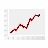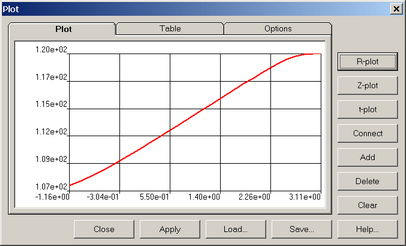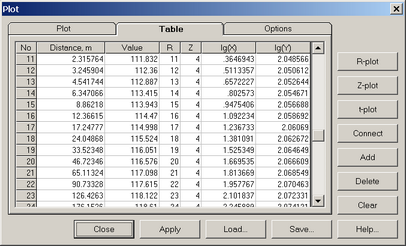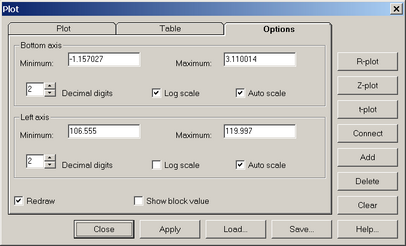﻿ Numerical modeling (ANSRADIAL) > Model editing > Graphic editor > Plot construction

# Plot construction

The "Plot" dialog box allows plotting time- and distance-drawdown plots.- call button in the graphic editor window."Plot" dialog boxes.

Explanatory table

 "Plot" tab Plot window "Table" tab Table display of the plot window "№" column Number of the points on the plot "Distance" column Distance from the first point on the plot. For time-drawdown plots, corresponds to time "Value" column Parameter value at the selected point "R" column The block (or node) number along the radial direction in which the value is given "Z" column The number along the vertical direction of the block (or node) for which the value is specified "lg(X)" column Decimal logarithm of the abscissa (time or distance) "lg(Y)" column Decimal logarithm of the ordinate "Options" tab Options for displaying the plot "Bottom axis" and "Left axis" frames Displaying coordinate axes "Minimum" text box The minimal value along the coordinate axis "Maximum" text box The maximal value along the coordinate axis "Decimal digits" text box The decimal number for coordinate axes values "Log-scale" option Displays coordinate axis in logarithmic scale "Auto scale" option The minimal and maximal coordinate values are default "Redraw" option Redraws the plot and clears the previous version "Show block value" option Draws a horizontal line on the plot indicating the value in the current block (or node) "R-plot" button Automatically plots a radial graphs for the specified parameter, including all the points/blocks in the selected layer "Z -plot" button Automatically plots a vertical graphs for the specified parameter, including all the points/blocks in the selected column "t -plot" button Automatically plots the time-drawdown plot for the current block (or node): for output file data only "Connect" button In case the new point of the plot ("Add" button) is more than one block (or node) apart from the previous one, the plot is automatically plotted through all intermediate nodes "Add" button Plots a new point for the selected block (or node) "Delete" button Deletes the last point from the plot "Clear" button Deletes all point to plot a new plot "Close" button Close the "Plot" dialog "Apply" button Applies the changes made at "Option" tab "Load" button Loads dat-file and display it on the plot "Save" button Saves plot as a dat-file